Archive
Special Issues
The Stability of High Order Max-Type Difference Equation
Applied and Computational Mathematics
Volume 5, Issue 2, April 2016, Pages: 51-55
Received: Apr. 6, 2016; Published: Apr. 7, 2016
Authors
Han Cai-hong, School of Mathematics and Statistics, Guangxi Normal University, Guilin, China
Li Lue, School of Mathematics and Statistics, Guangxi Normal University, Guilin, China
Tan Xue, School of Mathematics and Statistics, Guangxi Normal University, Guilin, China
Article ToolsAbstractPDF (721KB)HTML
Abstract
In this paper, we investigate the stability of following max-type difference equation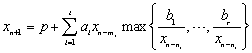, where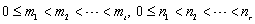, with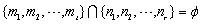,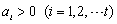,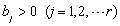and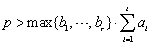, the initial values are positive. By constructing a system of equations and binary function, we show the equation has a unique positive equilibrium solution, and the positive equilibrium solution is globally asymptotically stable. The conclusion of this paper extends and supplements the existing results.
Keywords
Difference Equations, Positive Solution, Convergence, Globally Stable
Han Cai-hong, Li Lue, Tan Xue, The Stability of High Order Max-Type Difference Equation, Applied and Computational Mathematics. Vol. 5, No. 2, 2016, pp. 51-55. doi: 10.11648/j.acm.20160502.13
References

El-Metwally H, “Global behavior of an economic model,” Chaos Solitons & Fractals, 33(3), 2007, pp. 994-1005.

El-Metwally H, El-Afifi M M, “On the behavior of some extension forms of some population Models,” Chaos Solitons & Fractals, 36(1), 2008, pp. 104-114.

Zhou L, Honghua H U, Liang C, et al, “Research on difference equation model in traffic flow calculation,” Journal of Chang chun University of Science & Technology, 2014, pp. 117-123.

Huang C M, Wang W P, “Applications of difference equation in population forecasting model,” Advanced Materials Research, 2014, pp. 1079-1080.

Berenhaut K, Foley J, S. Stević S, “The global attractivity of the rational difference equation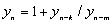,” Proceedings of the American Mathematical Society, 135, 2007, pp. 1133-1140.

Berenhaut K S, Stević S, “The behavior of the positive solutions of the difference equation,” J. Journal of Difference Equations and Applications, 12(9), 2006, pp. 909-918.

Berg L, Stević S, “Periodicity of some classes of holomorphic difference equations,” Journal of Difference Equations and Applications, 12(8), 2006, pp. 827-835.

Iričanin B, Stević S, “Some systems of nonlinear difference equations of higher order with periodic solutions,” Dynamics of Continuous Discrete and Impulsive Systems Series, 13A (3-4), 2006, pp. 499–507.

Iričanin B, Stević S, “Eventually constant solutions of a rationa ldifference equation,” Applied Mathematics and Computation, 215, 2009, pp. 854-856.

Elabbasy E M, El-Metwally H A, Elsayed E M, “Global behavior of the solutions of some difference equations,” Advances in Difference Equations, 28(2), 2011, pp. 683-689.

Elsayed E M, Iričanin B, Stević S, “On the max-type equation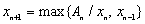,” Ars Combinatoria, 95, 2010, pp. 187-192.

Stević S, “Global stability of a max-type difference equation,” Applied Mathematics & Computation, 216(1), 2010, pp. 354–356.

Sun T X, Xi H J, Han C H, “Dynamics of the max-type difference equation,” Journal of Applied Mathematics and Computing, 2012 (1-2), 2012, pp. 173-180.

Stević S, “On a symmetric system of max-type difference Equations,” Applied Mathematics and Computation, 219(15), 2013, pp. 8407-8412.

Stević S, “On some periodic systems of max-type difference equations,” Applied Mathematics and Computation, 218, 2012, pp. 11483–11487.

Amleh A M, Georgiou D A, Grove E A, Ladas G, “On the recursive sequence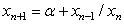,” Journal of Mathematital Analysis and applications, 233(2), 1999, pp. 790-798.

Fan Y, Wang L, Li W, “Global behavior of a higher order nonlinear diference equation,” Journal of Mathematital Analysis and applications, 299(1), 2004, pp. 113-126.

Sun T X, He Q L, Wua X, Xi H J, “Global behavior of the max-type difference equation,” Applied Mathematics and Computation, 248, 2014, pp. 687-692.

Liu W P, Stević S, “Global attractivity of a family of non-autonomous max-type difference equations,” Applied Mathematics and Computation, 218(11), 2012, pp. 6297-9303.

Grove E A, Ladas G, Periodicities in Nonlinear Difference Equations, Vol. 4, New York: Chapman& Hall/CRC Press, 2005, pp. 2.
PUBLICATION SERVICES